客服热线：133-4342-757513102219556•••# 安全鞋耐冲击试验机 压缩穿刺试验机 钢球冲击试验机 冲击试验机

• 面议

CJK-1459

13102219556 13102219556
• 发货地  天津
• 供货总量  100 台

• 暂无产品目录
 有效期至长期有效 更新时间2020-05-09 10:37 访问次数544

r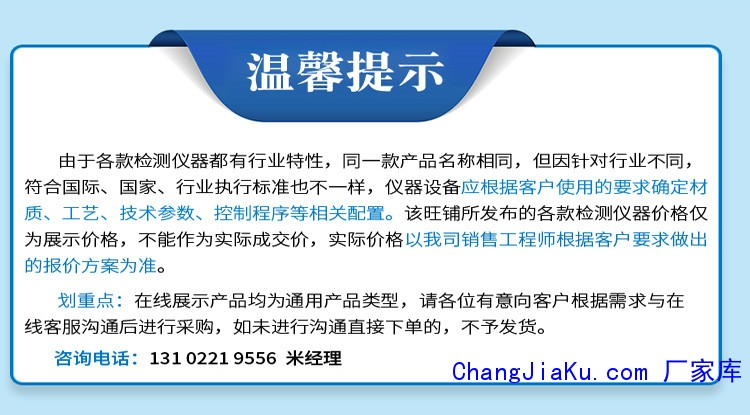r

r

r
r

r

r 一、主要用途: r

r

r 本设备根据安全鞋新国标GB/T20991-2007《个体防护装备 鞋的测试方法》中的标准研制，主要用于检测安全鞋鞋头盖的抗压缩性和钢中板的耐穿刺性能。 r

r

r 二、仪器特征： r

r

r 1、测定鞋头盖的抗压缩性，以及钢中板的耐穿刺性，防止因鞋子安全性能不够而造成作业者在工作场所穿着时受到意外的伤害。 r

r

r 2、此试验机具有调速功能，测试速度可根据客户要求任意调节，而且具有kgNlb三种单位可任意切换功能，并带有微型打印机，可将试验结果打印出来。 r

r

r 3、具有防第二次冲击功能，安全杜绝第二次冲击对试验所产生之误判。 r

r

r  三、主要技术指标： r

rr r r r r r r r r r r r r r r r r r r r r r r r r r r r r r r r r r r r r r r r r r r r r r r r r r r r r r r r r r r r r r r r r r r
 r r 主机结构 r r r r 桌上型，可控制压缩速度，实时显示钢头之压缩应力值，位移等 r r r r 传感器容量 r r r r 20kN r r r r 力量分析度 r r r r 1/10000 r r r r 准确度 r r r r ±0.1% r r r r 显示系统 r r r r HY-VS中/英文控制器，直观操作方便 r r r r 电机 r r r r AC变频电机，变频器控制 r r r r 测试夹具 r r r r 压缩/穿刺夹具各一套 r r r r 压板硬度 r r r r 60HRC r r r r 穿刺钢钉 r r r r 直径为（4.5±0.05）mm,有一个截平的端，端头应有至少60HRC的硬度 r r r r 测试速度 r r r r 穿刺速度（10±3）mm/min，压缩速度（5±2）mm/min（5~500mm/min无极调速） r r r r 位移显示 r r r r 0.01mm r r r r 打印功能 r r r r 选配 r r r r 体积 r r r r 82*58*71cm r r r r 重量 r r r r 148kg r r r r 电源 r r r r AC220V50HZ r r r r 符合标准 r r r r ISOr 20344、EN12568、GB/T 20991-2007 个体防护装备 鞋的测试方法 r r
r

r
r

r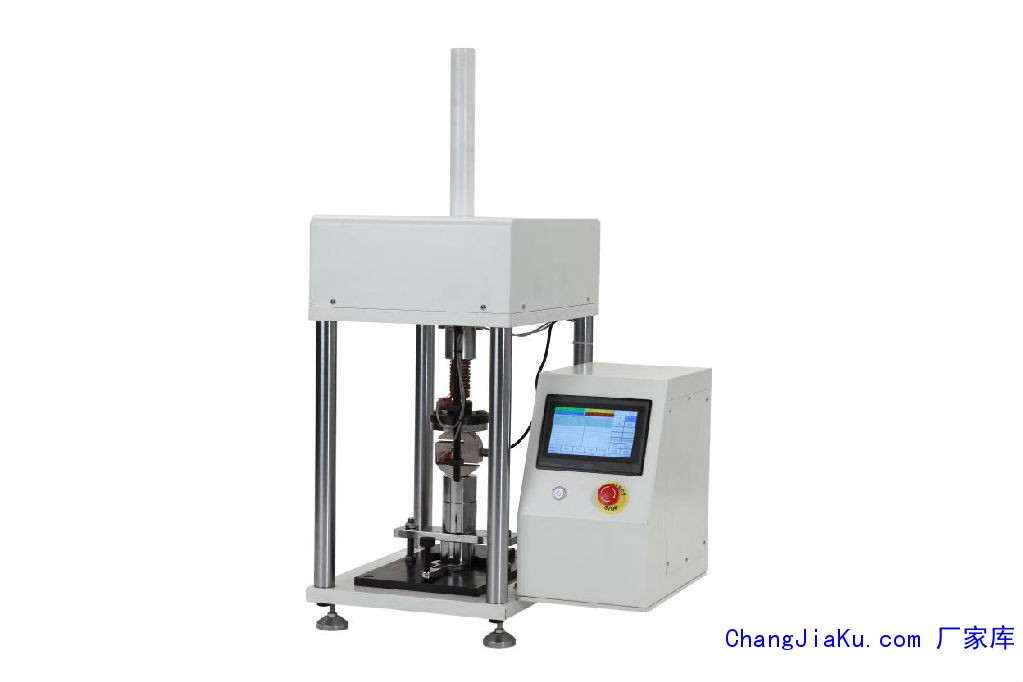r

r
r

r

r
r

r

r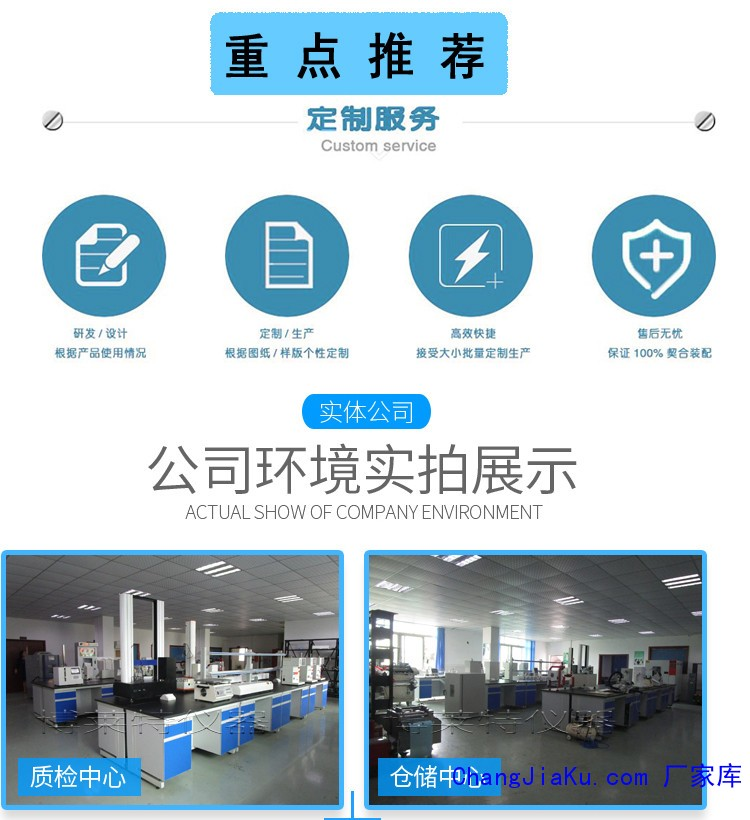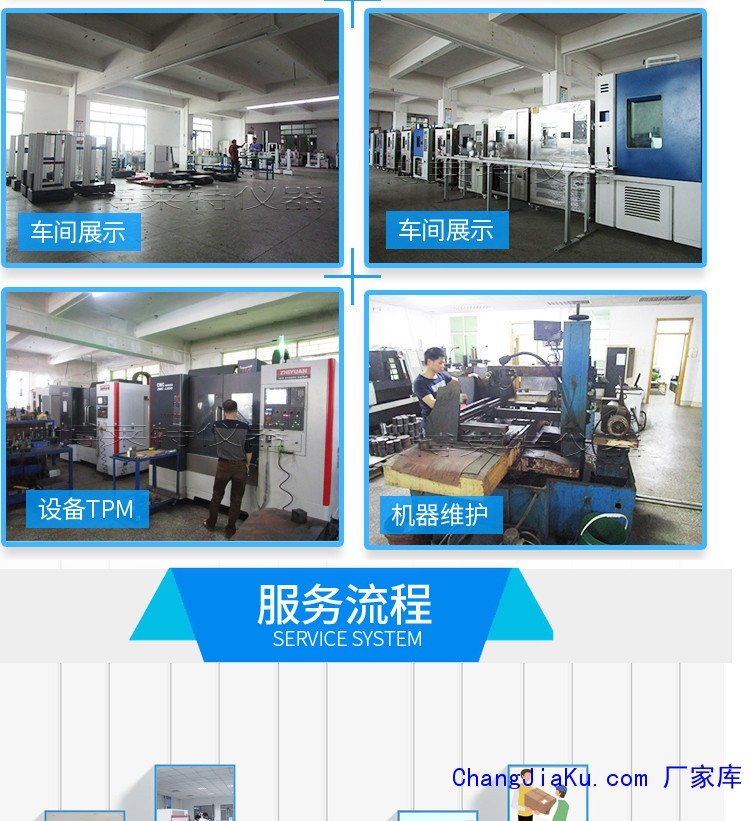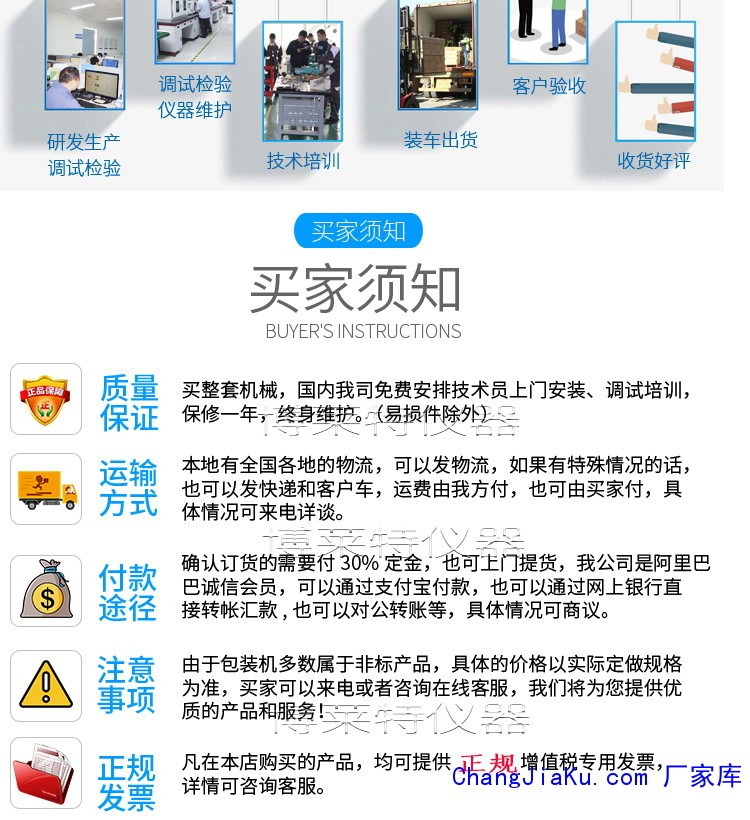r

## 全自动盐水浸泡试验机|全自动盐水浸泡试验BS EN ISO 8442 BS5577:1999 QB/T2174-2006 GB1506-2-1994★主要用于与食品接触制品与材料的盐水耐腐蚀性试验；海水浸泡试验**是将测试产品浸入到盐水中然后再停留在空气中，根据欧盟相关试验标准，保证每分钟升降三次。海水浸泡试验箱的做法分封闭式和开放式两种做法。结构材料：1.开放式海水浸泡试验机的盐水槽采用#304不锈钢制作,封闭式海水浸泡试验机的试验室采用8mmPVC板材制作。 试验室底部设有排水口，箱体外部由阀门来控制

## 编织袋电子拉力试验机 编织袋试验机 编制材## 厂家直销手动压滚 辗压滚轮 手动辗压滚轮、## 刀具试验机/菜刀专用检测仪器/水果刀锋利度## 高精度眼镜检测仪器 眼镜测试仪器　金属镜## 安全鞋皮革耐磨试验机 DIN磨耗试验机 辊筒## 生产供应 鞋底弯折试验机 大底弯曲试验机 E## 盐水浸泡试验机/小型刀具耐腐蚀试验机/人工BS EN ISO 8442 BS5577:1999 QB/T2174-2006 GB1506-2-1994★主要用于与食品接触制品与材料的盐水耐腐蚀性试验；海水浸泡试验**是将测试产品浸入到盐水中然后再停留在空气中，根据欧盟相关试验标准，保证每分钟升降三次。海水浸泡试验箱的做法分封闭式和开放式两种做法。结构材料：1.开放式海水浸泡试验机的盐水槽采用#304不锈钢制作,封闭式海水浸泡试验机的试验室采用8mmPVC板材制作。 试验室底部设有排水口，箱体外部由阀门来控制

## 浙江温州笔套拉力试验机、上海笔套拉力测试## 电子多功能试验机 汽车配件拉力试验机 标准